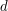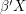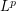## Optimal quantization applied to sliced inverse regression

 Title Optimal quantization applied to sliced inverse regression Publication Type Journal Article Year of Publication 2011 Authors Romain Azaïs, Anne Gégout-Petit, and Jérôme Saracco Keywords optimal quantization, reduction dimension, semiparametric regression model, sliced inverse regression (SIR) Abstract In this paper we consider a semiparametric regression model involving a-dimensional quantitative explanatory variableand including a dimension reduction ofvia an index. In this model, the main goal is to estimate the Euclidean parameter and to predict the real response variableconditionally to. Our approach is based on sliced inverse regression (SIR) method and optimal quantization in-norm. We obtain the convergence of the proposed estimators of and of the conditional distribution. Simulation studies show the good numerical behavior of the proposed estimators for ﬁnite sample size.